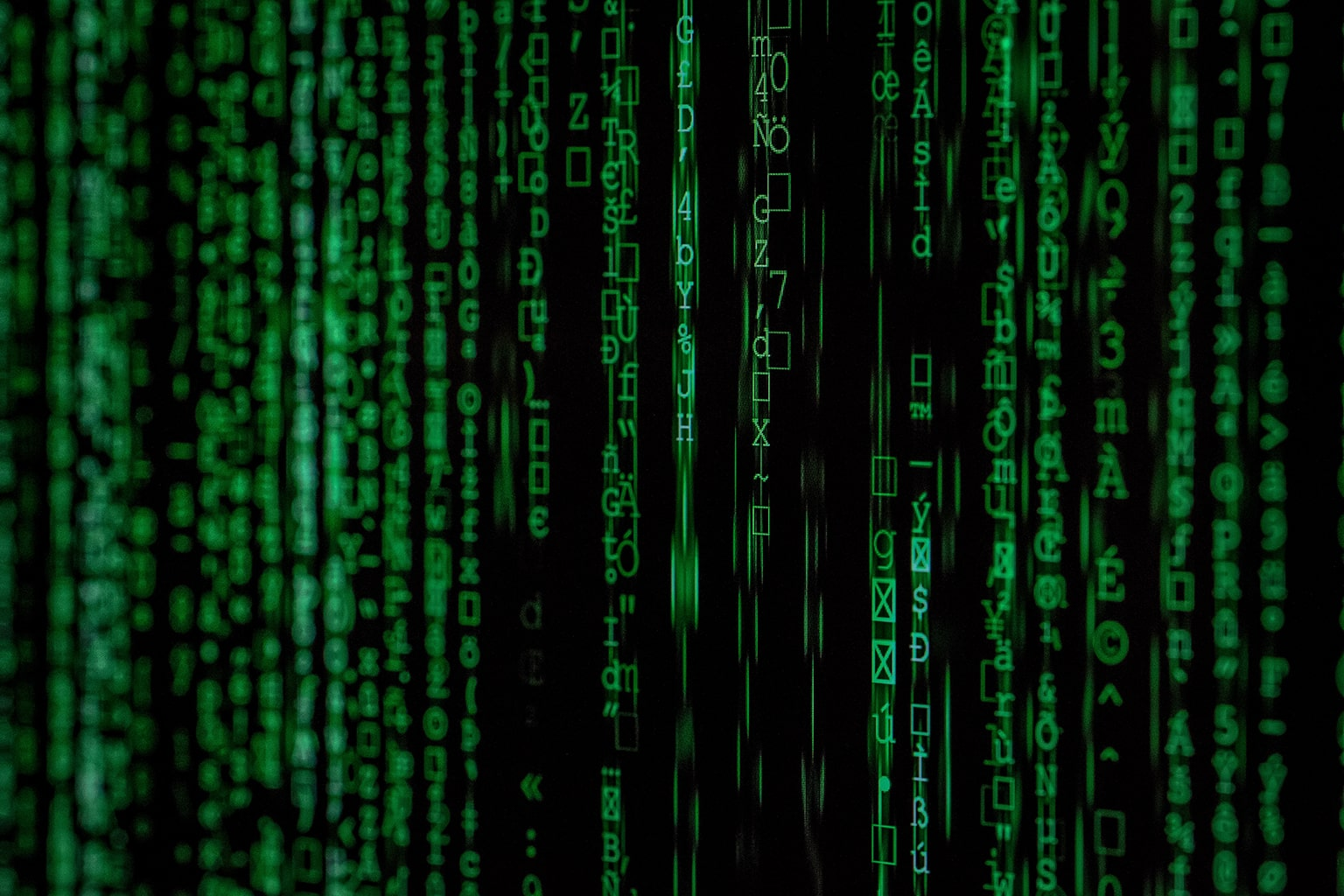Echelon Form of a matrix is used to solve a linear equation by converting a complex matrix to a simple matrix. A matrix is in an Echelon Form if it satisfies some conditions which we’ll discuss in this post. We must know how to convert a matrix into Echelon Form and simplify our matrix for further linear algebra operations.

## What is Echelon Form

A matrix is in an Echelon Form when it satisfies the following conditions:

• The first non-zero element in a row is 1. This entry is known as a pivot or leading entry.
1
2
3
0
1
2
0
0
1
1
0
2
0
0
1
0
0
0
1
2
3
0
2
3
0
0
1
• Each pivot in a column is the right side of the pivot column in the previous row.
1
2
0
1
1
0
0
1
1
2
3
0
1
2
0
1
5
• A row with all zeros should be below rows having a non-zero element.
1
2
3
4
0
1
2
3
0
0
1
2
0
0
0
0
1
2
3
4
0
0
1
0
0
0
0
1
0
0
0
0
1
2
3
4
0
1
2
3
0
0
0
0
0
0
1
0

This process of converting a matrix to echolon form is known as Gaussian elimination process. If you apply Gaussian Elimintation on rows then it’s Row Echelon Form and if apply on columns then its Column Echelon Form. We can say that inverse of Row Echelon Form is Column Echelon Form.

## Reduced Row Echelon form

This is a special form of a row echelon form matrix. So A row echelon form is reduced row echelon form if it satisfies the following condition:

• A pivot or leading entry 1 in the row will be the only non-zero value in its columns. So all other values in the same column will have zero value.
1
0
3
0
0
1
2
0
0
0
0
1
0
0
0
0

So note that all other values are zero in the same column which has leading 1 (red highlighted).

Every matrix has a unique reduced row echelon form and helps to solve a linear system easily.

## Convert to Row Echelon Form

We can convert any matrix into an row echelon form by applying multiple elementary operations. There are 3 main elementary operation as describe below:

• Interchange two Rows or Columns
4
5
6
7
1
2
3
4
8
9
10
11
0
0
0
0

R1 <> R2

1
2
3
4
4
5
6
7
8
9
10
11
0
0
0
0
• Multiply each value in the row or column by a non-zero value
1
2
3
4
5
6
7
8
9

(2R1 –> R1)

2
4
6
4
5
6
7
8
9
• Multiply each value in the row or column by a non-zero value and Adding to another row.
1
2
3
4
5
6
7
8
9

(-2R1 + R2 –> R2)

1
2
3
2
1
0
7
8
9

This process is known as Gaussian Elimintation process. And further converting a row echelon form to reduced row echelon form is known as Gauss‐Jordan Elimination process. Both process are very useful to solve a linear equations system. Let’s understand this by an example blew.

## Solving Linear Equations

Let’s consider two linear equations and find out x and y value using the gaussian elimination method.

X + Y = 2 and X+ 2Y = 3

Step 1 – Create a coefficient matrix. This is a matrix where each row represents an equation and columns values are coefficient value for each variable.

1
1
1
2

Step 2 – Create a constant matrix. This is a column matrix where each value represents the solution of the equation.

2
3

Step 3 – Create an augmented matrix which is a combined form of the coefficient matrix and constant matrix.

1
1
2
1
2
3

Step 4 – Convert this augmented matrix into row echelon form using elementary operations.

1
1
2
1
2
3

(R2 – R1 -> R2)

1
1
2
0
1
1

note that resultant matrix is in row echelon form so now rewrite the matrix as an equations.

1*X+1*Y = 2 and 0*X + 1*Y = 1

So Y=1 according to second equation and subsitute this value in the first equation to find the value of X. so the solution to the system of equations is (1,1).

I used a very simple example so you can understand it easily.